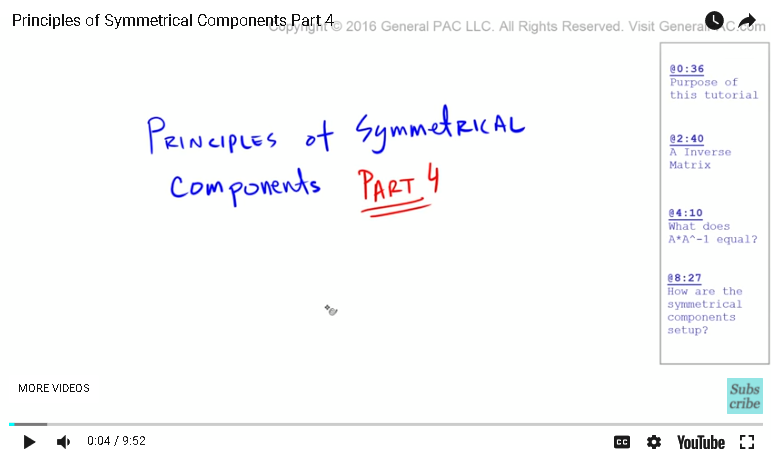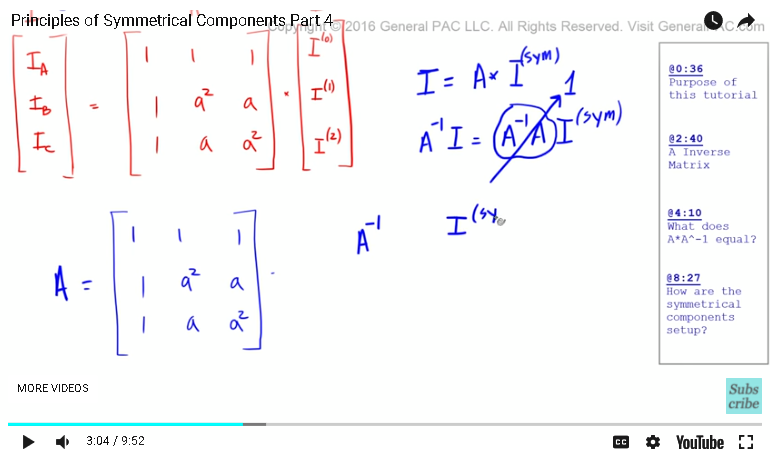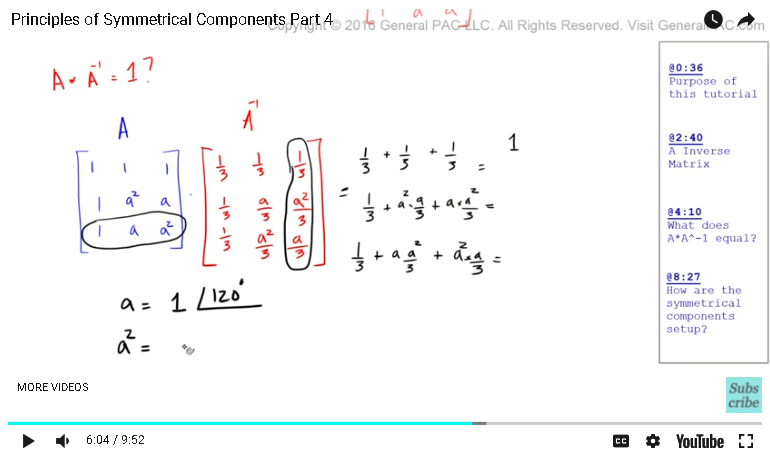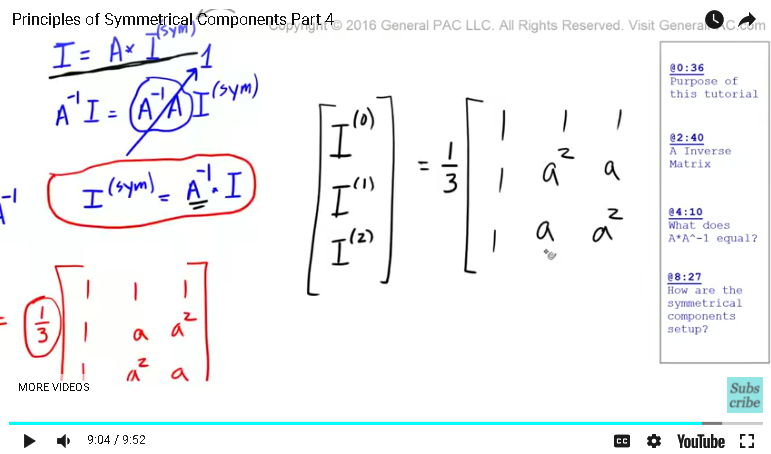Resources Section

Web Application for Symmetrical Componets: http://personal.strath.ac.uk/steven.m.blair/seq/

Do you have a question? Click on the "Questions & Answers forum" and ask away!

This video was brought to you by GeneralPAC.com, making power systems Intuitive, Open and Free for Everyone, Everywhere. Consider subscribing and supporting through patreon.com/GeneralPAC. This is a mechanism for you to support us financially so we can continue making high quality power system video tutorials. Our corporate sponsor for this topic is AllumiaX.com from Seattle, Washington. Contact them for industrial and commercial power system studies.

Principles of symmetrical component part four. In the previous part, we learned how to decompose the positive, negative, and zero sequence components to get the system phasors. We came up with this matrix form, which represented the system phasors in this column right here. So we'll call this "I." And then we had this matrix here, which we defined as, "A." And then we had the symmetrical component. So the symmetrical components were zero sequence component, positive sequence component, and negative sequence component. So in this video tutorial in part four, we want to know how to derive the symmetrical component, the zero sequence, positive sequence, and negative sequence component, from the system phasors directly.So if we had an unbalanced set of three phasors, something like that, where this was IA, this was IB, and this was IC, we want to know what are the positive sequence component, the negative sequence component, and the zero sequence component for this unbalanced set. What we're essentially saying is given this, this is given now, we want to calculate this.

So let's get started. The concept behind calculating the symmetrical component from the unbalanced system phasors is very simple. It's not hard, it's not difficult, but it needs to be understood from a fundamentalist level. So this is kind of like an algebraic equation where we have I and we have this A matrix right here and we're multiplying that by the symmetrical component. So if this was an algebraic equation and we wanted to calculate this here, what would we have to do? So if this was given, this was given, we wanted to calculate this. Well, that's pretty simple, right? It's equal to the system phasors divided by A. Pretty straight forward. But my friends, this is a linear algebraic equation and so using this same techniques won't exactly work.

So how do we calculate this guy right here given that we have this and we have this information? This is where the principles of linear algebra comes into play, but we will do is to learn it enough to understand the underlying principles behind this concept. So the idea is that if we can calculate the inverse of the A matrix, A inverse, then what we could do is multiply both sides of the equation by the inverse of the A matrix. And once we do that, this term right here, A times A inverse, will just equal to one. And so what we get is the I symmetrical component is going to equal A inverse times the system phasors. So that, my friend, is what the solution is and we'll talk more about this in just a little while.So let's first define what A inverse is. It turns out that if you take the A and we find the inverse of A, it turns out that that is equal to one third times this matrix here. So you can see that there is sort of a symmetry between A and A inverse. What we need to prove is A times A inverse. Does that equal to one? Well, let's find out. So that's A and then A inverse is one third here, one third here. Okay. So this is A inverse. And the one thirds are pretty straight forward to figure out. See this one third constant here? This one third constant would need to be multiplied by each element within this matrix. Okay. So let's find out what that is equal.

What we have to do is multiply this row here with this column here. Okay. So when we do that, what we get is one times one third is one third plus one times one third is one third plus one times one third is one third. Here, we just get one. Okay. So now the second row here, that is equal to the second row of the A matrix multiplied by the second column of the A inverse matrix. So one times one third is one third plus A squared times A divided by three, A squared times A divided by three, and we'll figure out what that means later, plus A times A squared divided by three, plus A times A squared divided by three. What does that equal? We'll get to that in just a little while. And to figure out what this third row is, we have to multiply the third row of the A matrix by the third column of the A matrix and that is equal to one third, one times one third is one third, A times A squared divided by three, so A times A squared divided by three, plus A squared times A divided by three, so A squared here. Okay.

So the question is what is these terms here? And to understand this, it's very simple. In the past, we defined A as one at the angle of 120 degrees and A squared, or A superscript two, as one at the angle of 240 degrees. So when we multiply A times A squared, what we're effectively doing is we're multiplying the magnitudes together and we're adding the angles. So we're going to multiply. When we multiply two phasors, we multiply the magnitudes and then add the angles. 120 plus 240 degrees. And that is equal one at 360 degrees. Now 360 degrees is the same thing as saying zero degrees. What this effectively means is that A times A squared is equal to one. So A times A squared is equal to one, which means that this right here, this term and this term, both of those terms multiplied together is equal to one. So what we have is one third plus one times one third plus one times one third. Well that is just equal to one. And then here, we have one third plus A times A squared that is equal to just one. So one third plus one times one third plus one times one third and that is also equal to one.So here what we just proved is that A times A squared simply equals one. So this is what we proved by going through this particular example. So if we have this equation that's defining the symmetrical component breakdown where the system phasors is equal to A times the symmetrical component. Well, if we wanted to solve for the symmetrical component, we can just multiply both sides of the equation by A inverse. And when we do that, A inverses times A, well that just equals one and then we have to multiply the left hand side of the equation by A inverse, which means that the symmetrical component is simply equal to A inverse times the system phasors. And so here is what we get.

So the symmetrical component were set up like this where we had the zero sequence component first, the positive sequence component second, and the negative sequence component third. Well, that is equal to A inverse, so A inverse is here, so remember we said that A inverse just one third is brought out as a coefficient and then we have one here, one here, one here, one here, one here, and then we have this sort of symmetry where we start with A squared and then we end with A squared. And then we multiply that by our system phasors, which is simply IA, IB, and IC. And there you have it. So this right here is our solution.In part four B, we'll give an example of this method so it can be solidified. This video was brought to you by GeneralPac.com. Making power systems intuitive. Now if you have any questions, please go ahead and click on the forum link on the bottom of this video and you can ask away on this forum. If you haven't subscribed already, please go ahead and click on the subscribe button on the bottom right corner of the screen to stay connected with this channel.

Thank you.

Greetings from the GeneralPAC Team!

We make high-quality Power Systems Video Tutorials on complex topics that are free and open to everyone!  Thank you so much for supporting us through Patreon so can continue doing good and valuable work.

What is Patreon and why do we use it?

Patreon is a fantastic portal that allows our fans and community to make monthly contribution (like Netflix subscription) so we can continue creating high-quality power systems video tutorials. In return, you get access to incredible perks like voting on future topics, getting your questions answered, access to VIP Q/A webinars with the creators of GeneralPAC, and much more! We THANK YOU for supporting us

Why do we need your support?

An incredible amount of time and effort is needed to develop high-quality video tutorials. Each video (Part 1 for example) takes approximately 10 hours to complete which includes learning the concept ourselves, brainstorming creative ways to teach and explain the concepts, writing the script, audio recording, video recording, and editing. It's no wonder why Hundreds-of-Thousands of people have watched, liked, subscribed, and left positive comments on Youtube channel. Your support truly makes all the difference.